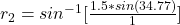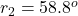## A ray of light strikes the midpoint of one face of an equiangular (60°–60°–60°) glass prism (n = 1.5) at an angle of incidence of 39.8°. (a)

Question

A ray of light strikes the midpoint of one face of an equiangular (60°–60°–60°) glass prism (n = 1.5) at an angle of incidence of 39.8°. (a) Trace the path of the light ray through the glass, and find the angles of incidence and refraction at each surface.

in progress 0
6 months 2021-07-22T03:15:13+00:00 1 Answers 17 views 0

a

The path of light ray through the glass is shown on the first uploaded image

First surface:

Angle of  incidence is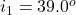Angle of  refraction  is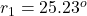Second surface:

Angle of  incidence is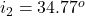Angle of  refraction  is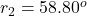b

Since the angle of incidence is equal to the angle of reflection

Then at the first surface the angle of reflection  is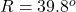And at the first surface the angle of reflection  is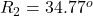Explanation:

From the question we are told that

The angle of incidence isThe refractive index of the prism is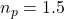The angle of the prism is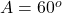The path of light ray through the glass is shown on the first uploaded image

For the first surface of the prism

According to Snell’s law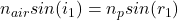The refractive index of air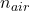has a constant value of  1

Now making  the angle of refraction at the first surface of the prism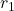the subject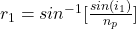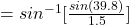For the second  surface of the prism

looking at the diagram on the first uploaded image the angle of incidence is mathematically evaluated  as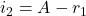Substituting values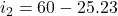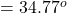According to Snell’s law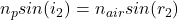Now making  the angle of refraction at the second surface of the prism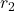the subject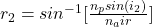Substituting values into the equation